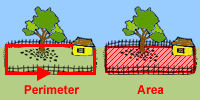# Perimeter and Area of Polygons

Perimeter and area of polygons are introduced in this unit. Perimeter of polygons and perimeter of regular polygons are covered. The difference between perimeter and area is explained. Try our sample lessons below, or browse other units.Perimeter & Area Description To understand the concept of perimeter. To find perimeter of triangles and perimeter of regular polygons. To express perimeter using linear units. Area of Rectangles To find the area of squares and rectangles. To contrast these concepts with perimeter. Square units are used. Area of Parallelograms To find the area of parallelograms using the proper formula. Area of Triangles To find the area of different types of triangles using the proper formula. Area of Trapezoids To find the area of different types of trapezoids using the proper formula. Practice Exercises To complete 10 additional exercises as practice. To assess students' understanding of all concepts learned in this unit. Challenge Exercises To solve 10 additional problems that challenge students' understanding of all concepts learned. Problems are drawn from real-life situations. To hone students' problem-solving skills. Solutions To review complete solutions to all exercises in this unit. Includes the problem, step-by-step solutions, final answer and units for each exercise. Learning Objectives Learning Topics: Perimeter of polygons, length, width, base and height, Area of rectangles, parallelograms, triangles and trapezoids.